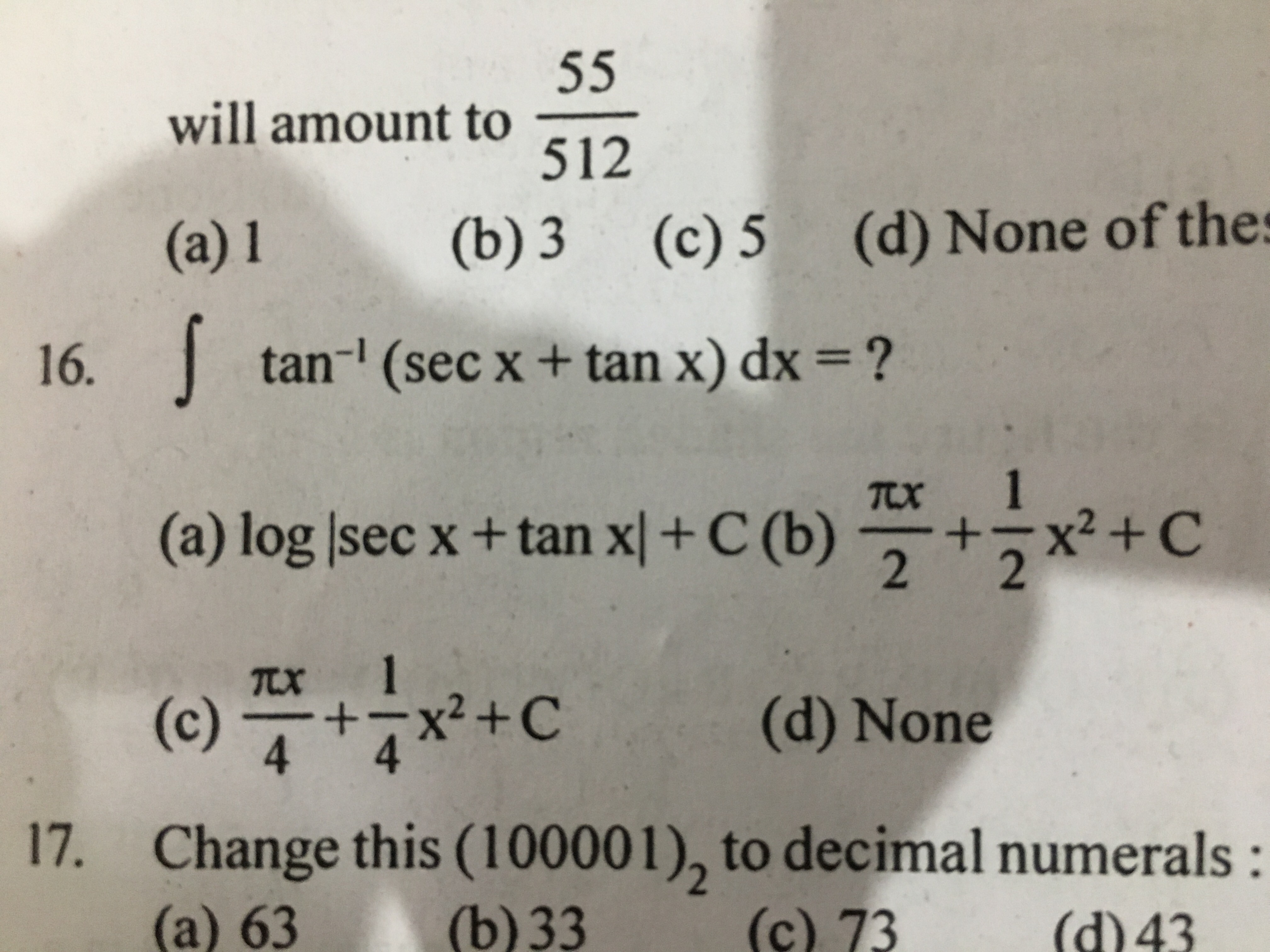# Can i know the answer of ques no. 16 bcoz i am little bit confuse in this question2 years ago

16)

I=tan^-1secx+tanx)dx

secx+tanx=1+sinx/cosx

1+sinx=(sinx/2+cosx/2)^2

cosx=(cosx/2-sinx/2)(cosx/2+sinx/2)

I=tan^-1(sinx/2+cosx/2)/(cosx/2-sinx/2)dx

=tan^-(tanpi/4+x/2)dx

=(pi/4+x/2)dx =(pi/4)x + x^2/4 +k